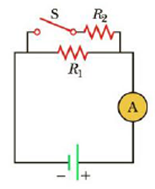Chapter 18.3, Problem 18.5QQ

Chapter
Section
Textbook Problem

In Figure 18.8, the current is measured with the ammeter on the right side of the circuit diagram. When the switch is closed, does the reading on the ammeter (a) increase, (b) decrease, or (c) remain the same?Figure 18.8 (Quick Quizzes 18.5 and 18.6)

To determine
The magnitude of current measured in the ammeter.

Explanation

Given Info: R1 , R2 are resistors in the circuit.

Explanation:

The current choose to flow from least resistive path. If the resistance of the path increases the flow of current in that path decreases.

When the circuit is closed, both the resistors are connected parallel to each other.

The effective resistance of the circuit decreases as the current find an additional way to flow that is through resistor R2 and reaches the ammeter

Still sussing out bartleby?

Check out a sample textbook solution.

See a sample solution

The Solution to Your Study Problems

Bartleby provides explanations to thousands of textbook problems written by our experts, many with advanced degrees!

Get Started

What are the moral implications of using ergogenic aids?

Understanding Nutrition (MindTap Course List)

Describe how Dalton obtained relative atomic weights.

General Chemistry - Standalone book (MindTap Course List)

Match the terms and definitions.

Human Biology (MindTap Course List)